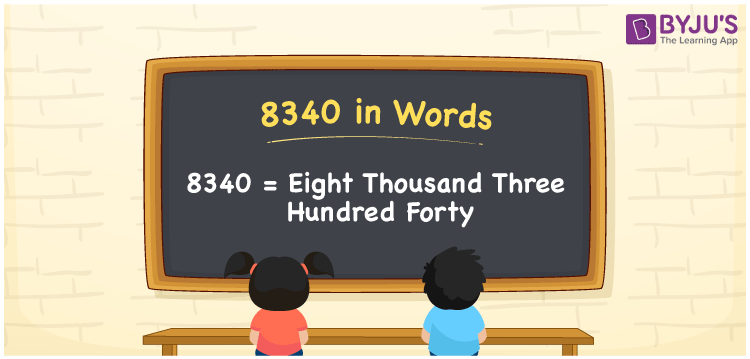# 8340 in Words

8340 in words is Eight thousand three hundred forty. The number 8340 has four digits and each digit’s place value helps to write the number name. As we know, 8340 is a cardinal number, since it helps to count things. For example, there are 8340 students in a school. This article will help you to write the number 8340 in words using the place value system in detail.

 8340 in Words: Eight Thousand Three Hundred Forty. Eight Thousand Three Hundred Forty in Numerical Form: 8340.

## 8340 in English Words## How to Write 8340 in Words?

The number 8340 has four digits and its place values are provided in the below table:

 Thousands Hundreds Tens Ones 8 3 4 0

The expanded form of 8340 is as follows:

= 8 × Thousand + 3 × Hundred + 4 × Ten + 0 × One

= 8 × 1000 + 3 × 100 + 4 × 10 + 0 × 1

= 8000 + 300 + 40

= Eight thousand three hundred forty

Hence, 8340 in words is eight thousand three hundred forty.

8340 in words – Eight thousand three hundred forty

Is 8340 an odd number? – No

Is 8340 an even number? – Yes

Is 8340 a perfect square number? – No

Is 8340 a perfect cube number? – No

Is 8340 a prime number? – No

Is 8340 a composite number? – Yes

## Frequently Asked Questions on 8340 in Words

Q1

### Write 8340 in words.

8340 in words is eight thousand three hundred forty.

Q2

### Simplify 8000 + 340, and express it in words.

Simplifying 8000 + 340, we get 8340. Hence, 8340 in words is eight thousand three hundred forty.

Q3

### Is 8340 a perfect square number?

No, 8340 is not a perfect square number.